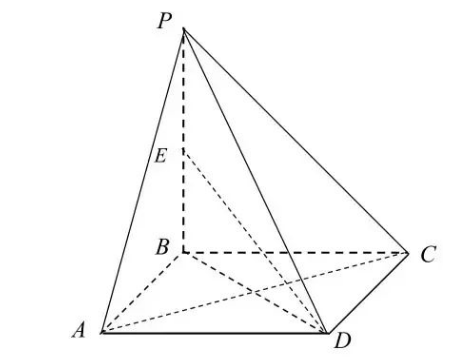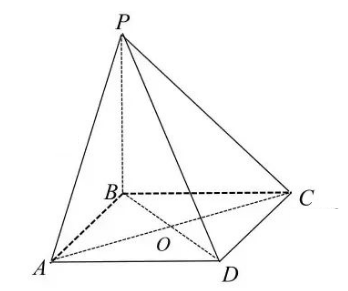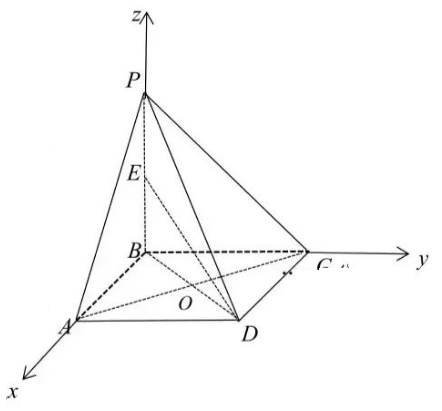(1) 证明: $A C \perp P D$;
(2) 若 $P B=2 A B=2$, 求直线 $D E$ 与平面 $P C D$ 所成角的正弦值.【答案】
(1) 证明: 连接 $A C$, 设 $A C$ 与 $B D$ 交点为 $O$, 连接 $P O$, 因为 $A B C D$ 为正方形, 所以 $A C \perp B D$, 因为 $P B \perp$ 平面 $A B C D$, 所以 $A C \perp P B$, 因为 $B D \cap P B=B$, 所以 $A C \perp$ 平面 $P B D$, 所以 $A C \perp P D$;(2)
(2) 解: